﻿ 基于生成对抗网络的机载遥感图像超分辨率重建
«上一篇文章快速检索 高级检索

 智能系统学报2020, Vol. 15Issue (1): 74-83  DOI: 10.11992/tis.2020020020

### 引用本文BI Xiaojun, PAN Mengdi. Super-resolution reconstruction of airborne remote sensing images based on the generative adversarial networks[J]. CAAI Transactions on Intelligent Systems, 2020, 15(1): 74-83. DOI: 10.11992/tis.202002002.### 文章历史

1. 中央民族大学 信息工程学院，北京 100081;
2. 哈尔滨工程大学 信息与通信工程学院，黑龙江 哈尔滨 150001

Super-resolution reconstruction of airborne remote sensing images based on the generative adversarial networks
BI Xiaojun 1, PAN Mengdi 2
1. School of Information Engineering, Minzu University of China, Beijing 100081, China;
2. Department of Information and Communication Engineering, Harbin Engineering University, Harbin 150001, China
Abstract: To solve the problem that the quality of airborne remote sensing images is susceptible to environmental impacts, super-resolution reconstruction is carried out. The existing super-resolution reconstruction methods for deep learning airborne remote sensing images has the problems of poor feature extraction capability, smooth edges of reconstructed images and difficulty in model training, the image reconstruction effect is enhanced to solve the above problems. The generative adversarial network is taken as the overall framework of the model. The dense residual block is used to enhance the feature extraction capability of the model, and jump connection is added to effectively extract the shallow and deep features of airborne remote sensing images. The Wasserstein-type generative adversarial network optimization model training is introduced. The method can effectively reconstruct airborne remote sensing images by 4 times, and has a gain of 2 dB or so in peak signal-to-noise ratio evaluation compared with other methods for comparison. The reconstructed airborne remote sensing images are clearer in vision, richer in details and sharper in edges. The experimental results show that the method effectively improves the model feature extraction ability, optimizes the training process, and the reconstructed airborne remote sensing image has better effect.
Key words: airborne remote sensing    super-resolution reconstruction    deep learning    residual in residual dense block    feature extraction    jump connection    Wasserstein    generative adversarial network

1 相关工作 1.1 超分辨率重建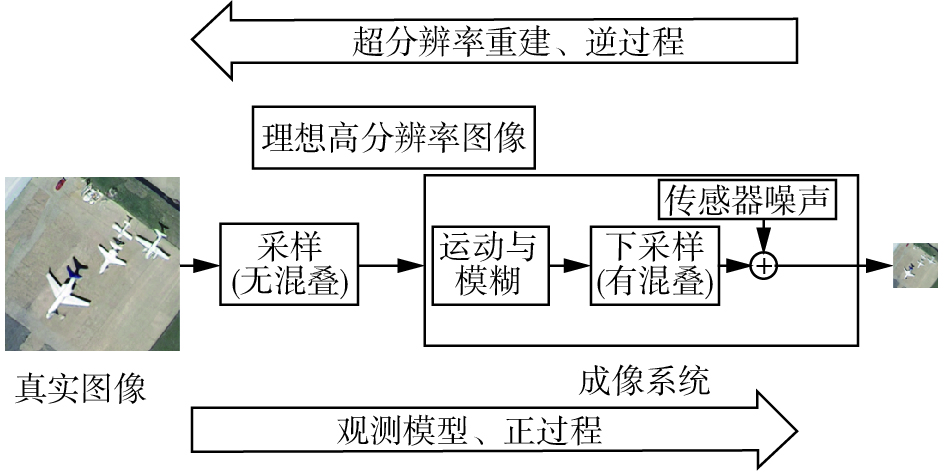Download: 图 1 成像正过程与超分辨率重建逆过程 Fig. 1 Positive imaging process and inverse process of super-resolution reconstruction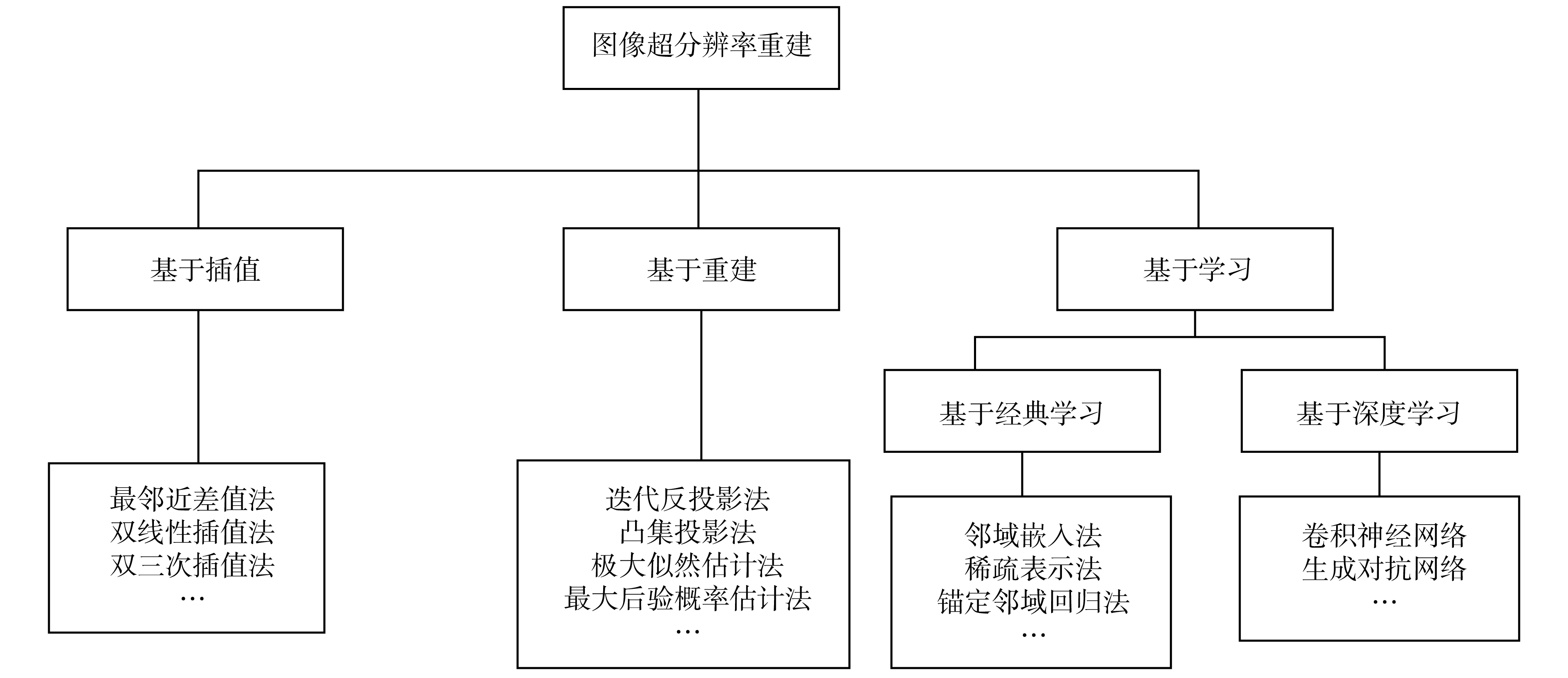Download: 图 2 图像超分辨率重建方法分类 Fig. 2 Classification of image super-resolution reconstruction methods
1.2 生成对抗网络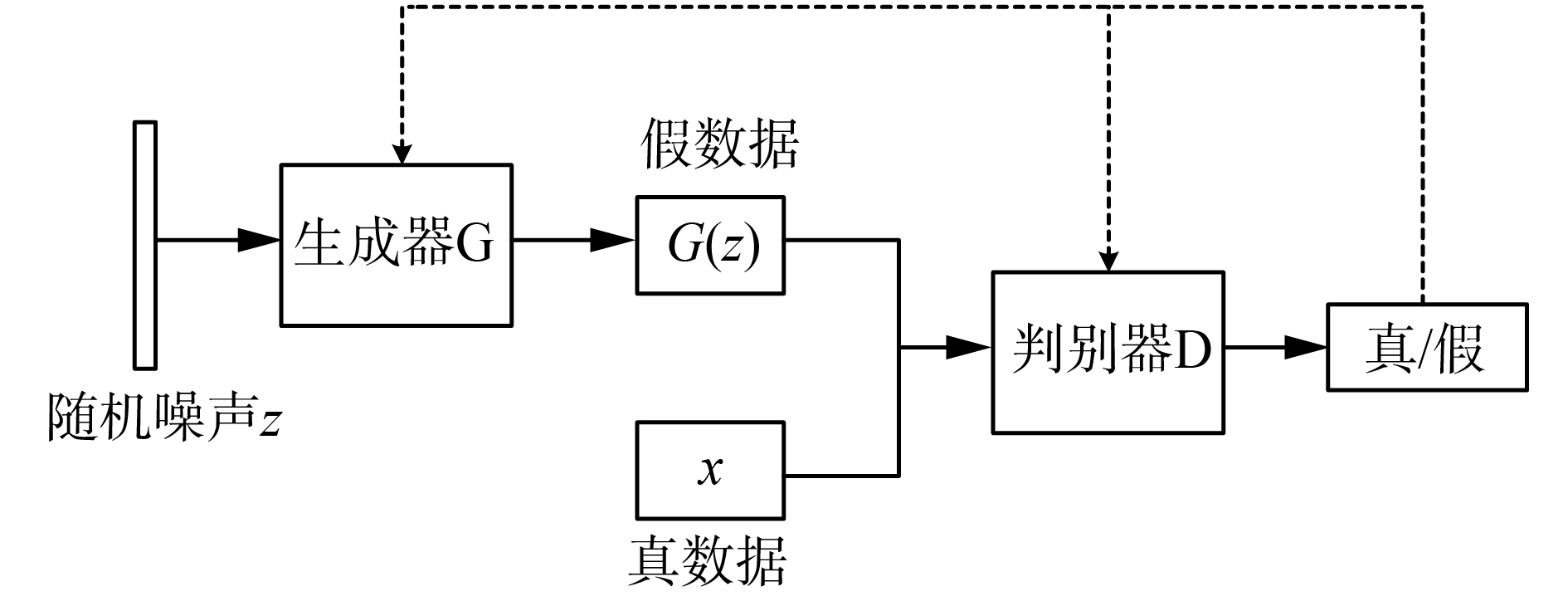Download: 图 3 生成对抗网络结构 Fig. 3 The structure of the generate adversarial network
1.3 沃瑟斯坦式生成对抗网络

 $W({P_{r,}}{P_g}) = \mathop {\inf }\limits_{\gamma \sim \prod ({P_{r,}}{P_g})} {E_{(x,y) \sim \gamma }}[\parallel x - y\parallel ]$

 ${L_{({P_{r,}}{P_g})}} = {E_{x \sim {P_r}}}[{f_w}(x)] - {E_{x \sim {P_g}}}[{f_w}(x)]$

 ${L_{\rm{G}}} = - {E_{x \sim {P_g}}}[{f_w}(x)]$
 ${L_{\rm{D}}} = {E_{x \sim {P_g}}}[{f_w}(x)] - {E_{x \sim {P_r}}}[{f_w}(x)]$

${L_{({P_r},{P_g})}}$ 越小，表示 ${P_r}$ ${P_g}$ 的Wasserstein距离越小，GAN训练得越好。

2 本文方法 2.1 网络结构设计Download: 图 4 网络整体结构 Fig. 4 Overall network structure
2.1.1 生成器网络的设计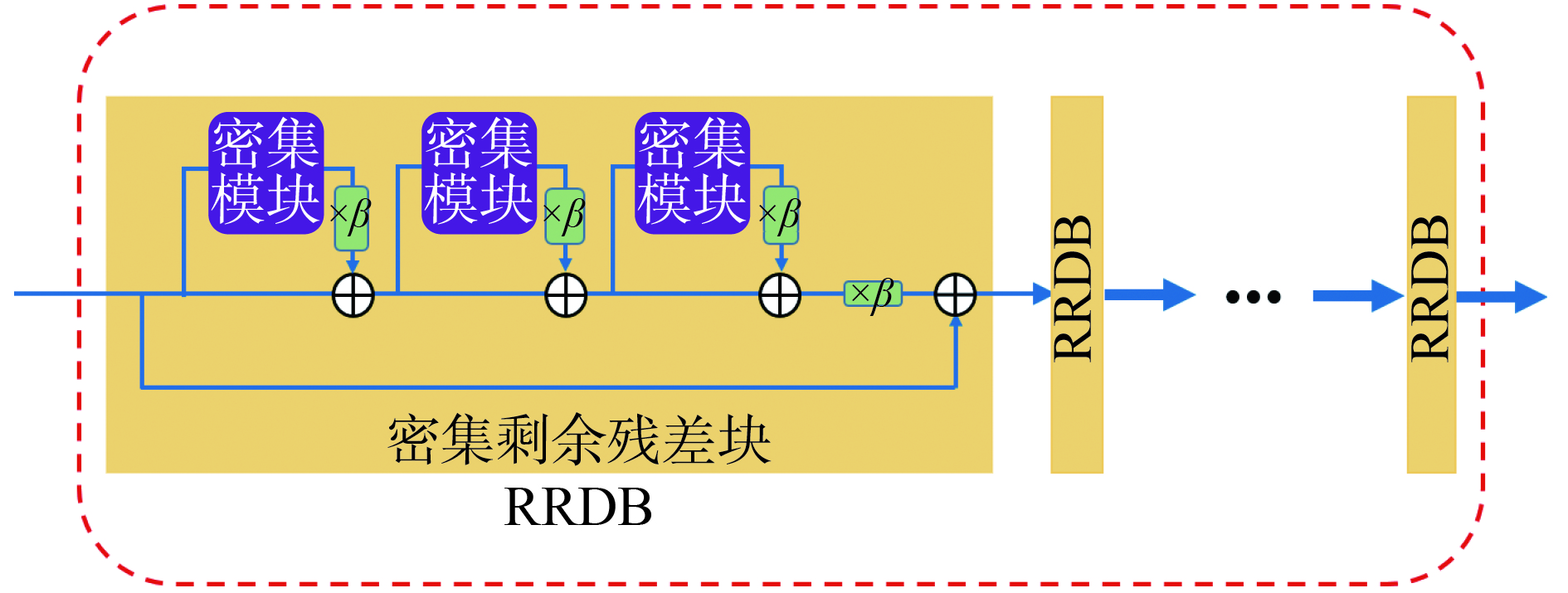Download: 图 5 特征提取模块 Fig. 5 Feature extraction block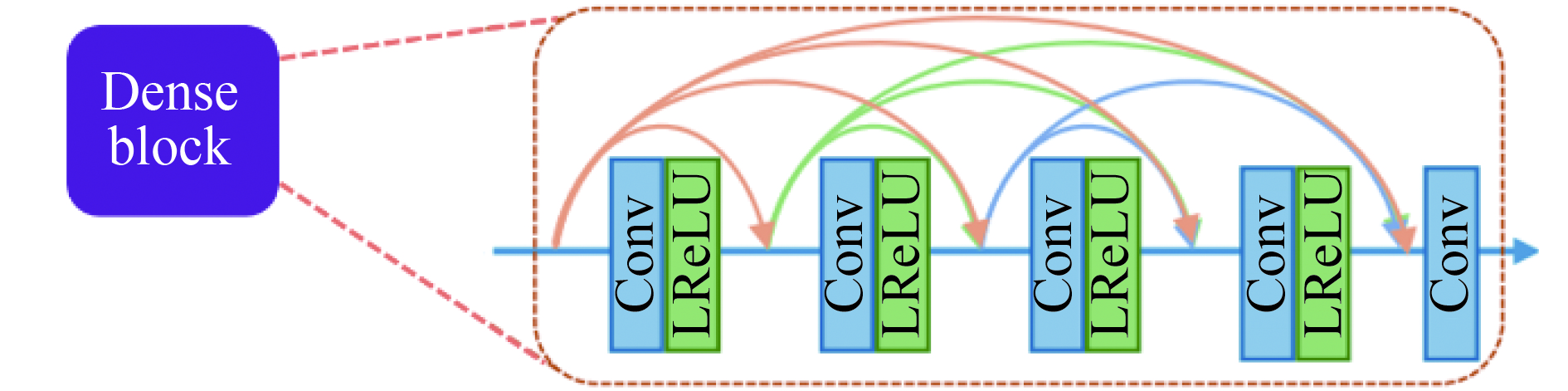Download: 图 6 密集模块 Fig. 6 Dense block

2.1.2 判别器网络的设计

2.2 损失函数与超参数设置

 ${L_{{\rm{percep}}}} = {L_{{\rm{content}}}} + {10^{ - 3}}{L_{{\rm{Gen}}}}$

 $\begin{array}{l} {L_{{\rm{content}}}} = {L_{{\rm{VGG}}/i,j}} = \dfrac{1}{{{W_{i,j}}{H_{i,j}}}}\displaystyle\sum\limits_{x = 1}^{{W_{i,j}}} {\displaystyle\sum\limits_{y = 1}^{{H_{i,j}}} {({\phi _{i,j}}{{({I^{HR}})}_{x,y}}} } -\\ \;\;\;\;\;\;\;\;\;\;\;\;\;\;\;\;\;\;\;\;\;\;\; {\phi _{i,j}}{({G_{{\theta _G}}}({I^{HR}}))_{x,y}}{)^2} \end{array}$

 ${L_{{\rm{Gen}}}} = - {E_{x \sim {P_g}}}[{f_w}(x)]$

 ${L_{\rm{D}}} = {E_{x \sim {P_g}}}[{f_w}(x)] - {E_{x \sim {P_r}}}[{f_w}(x)]$

2.3 评价指标的选取

 ${\rm{PSNR}} = 10 \times \lg \Bigg[\frac{{{{({2^n} - 1)}^2}}}{{{\rm{MSE}}}}\Bigg]$

 ${\rm{MSE}} = \frac{{\displaystyle\sum\limits_{i = 1}^M {\displaystyle\sum\limits_{j = 1}^N {({I_{ij}} - I_{ij}')} } }}{{M \times N}}$

3 实验与结果分析 3.1 数据集与实验环境表 1 实验环境配置 Tab.1 Experimental environment configuration
3.2 实验结果分析表 2 NWPU-RESISC45测试集的PSNR对比 Tab.2 PSNR comparison of NWPU-RESISC45 test setDownload: 图 7 NWPU-RESISC45的重建效果对比 Fig. 7 Comparison of reconstruction effect of test set NWPU-RESISC45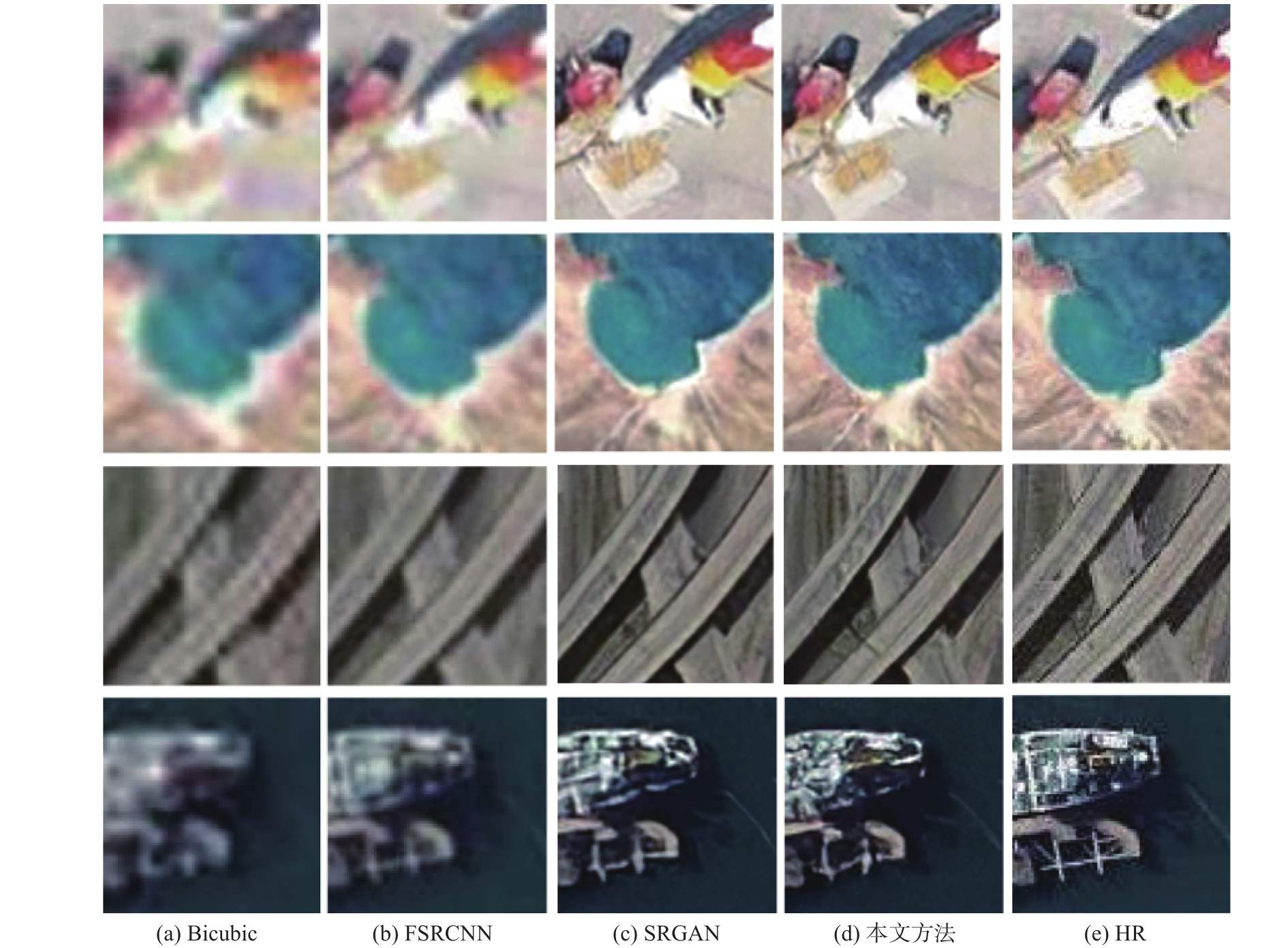Download: 图 8 局部放大效果对比 Fig. 8 Comparison of local magnification表 3 UCMerced_LandUse测试集的PSNR对比 Tab.3 PSNR comparison of UCMerced_LandUse test setDownload: 图 9 测试集UCMerced_LandUse的重建效果对比 Fig. 9 Comparison of reconstruction effect of test set UCMerced_LandUse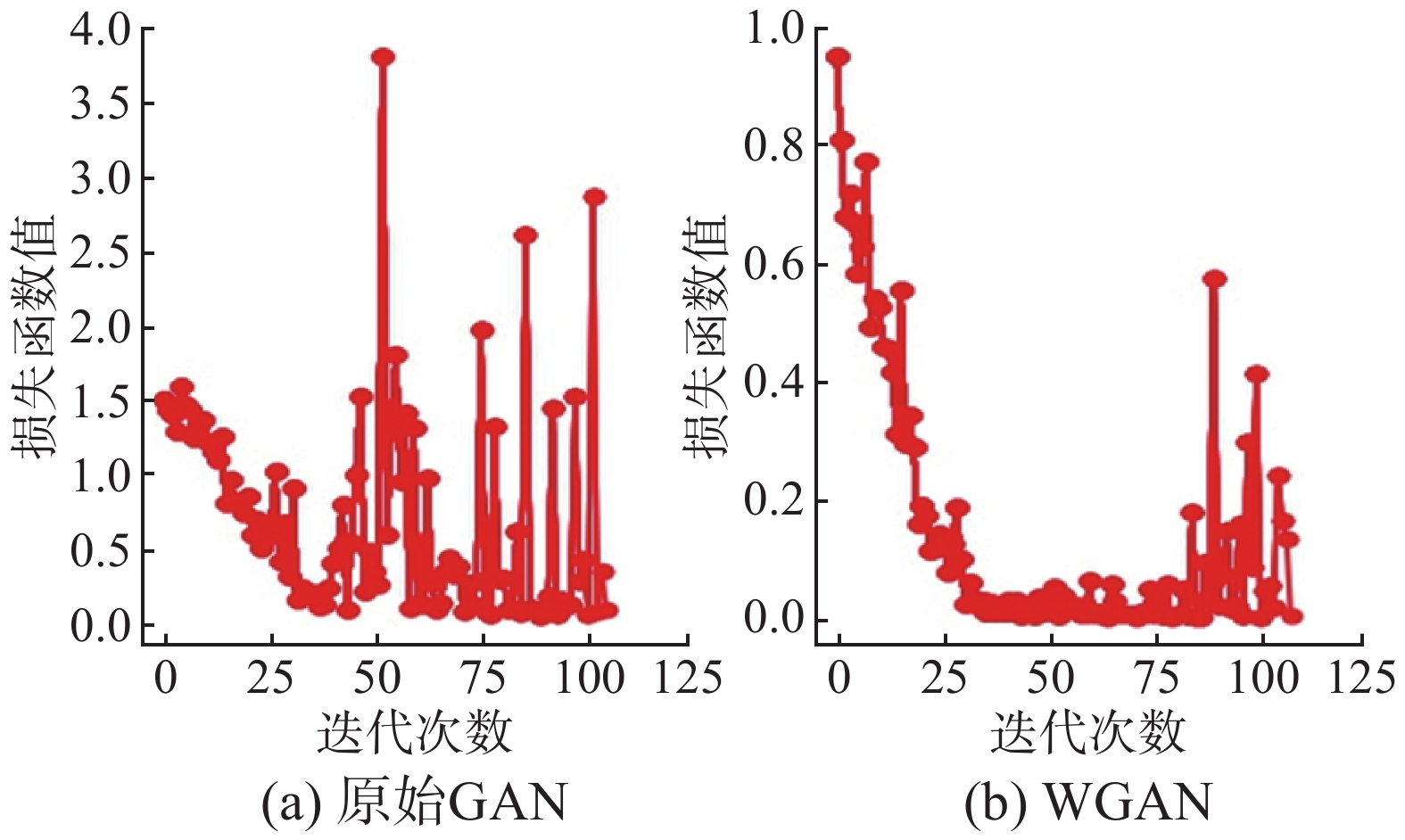Download: 图 10 判别器损失函数变化曲线对比 Fig. 10 Comparison of discriminant loss function curves
4 结束语

  赵英时. 遥感应用分析原理与方法[M]. 北京: 科学出版社, 2013. (0)  罗小波. 遥感图像智能分类及其应用[M]. 北京: 电子工业出版社, 2011. (0)  石爱业, 徐枫, 徐梦溪. 图像超分辨率重建方法及应用[M]. 北京: 科学出版社, 2016. (0)  DONG Chao, LOY C C, HE Kaiming, et al. Learning a deep convolutional network for image super-resolution[M]. FLEET D, PAJDLA T, SCHIELE B, et al. Computer Vision- ECCV 2014. Cham: Springer, 2014: 184−199. (0)  DONG Chao, LOY C C, TANG Xiaoou. Accelerating the super-resolution convolutional neural network[M]. LEIBE B, MATAS J, SEBE N, et al. Computer Vision- ECCV 2016. Cham: Springer, 2016: 391−407. (0)  KIM J, LEE J K, LEE K M. Accurate image super-resolution using very deep convolutional networks[C]//Proceedings of 2016 IEEE Conference on Computer Vision and Pattern Recognition. Las Vegas, NV, USA, 2016: 1646−1654. (0)  LEDIG C, THEIS L, HUSZÁR F, et al. Photo-realistic single image super-resolution using a generative adversarial network[C]//Proceedings of 2017 IEEE Conference on Computer Vision and Pattern Recognition. Honolulu, HI, USA, 2017: 105−114. (0)  ARJOVSKY M, CHINTALA S, BOTTOU L. Wasserstein GAN[J/OL]. (2017-01-26). https://arxiv.org/abs/1701.07875. (0)  石爱业, 徐枫, 徐梦溪. 图像超分辨率重建方法及应用[M]. 北京: 科学出版社, 2016. (0)  TSAI R Y, HUANG T S. Multiframe image restoration and registration. Advances in Computer Vision and Image Pro-cessing: JAI Press Inc., 1984, 317−339. (0)  高春波. 生成对抗网络的图像超分辨率重建[D]. 杭州: 浙江理工大学, 2019. GAO Chunbo. Image super-resolution using a generative adversarial network[D]. Hangzhou: Zhejiang Sci-Tech University, 2019. (0)  GOODFELLOW I J, POUGET-ABADIE J, MIRZA M, et al. Generative adversarial nets[C]//Proceedings of the 27th International Conference on Neural Information Processing Systems. Montreal, Canada, 2014: 2672−2680. (0)  IOFFE S, SZEGEDY C. Batch normalization: accelerating deep network training by reducing internal covariate shift[C]//Proceedings of the 32nd International Conference on Machine Learning. Lille, France, 2015: 448−456. (0)  MASS A L, HANNUN A Y, NG A Y. Rectifier nonlinearities improve neural network acoustic models[C]//Proceedings of the 30th International Conference on Machine Learning. Atlanta, GA, USA, 2013. (0)  LIM B, SON S, KIM H, et al. Enhanced deep residual networks for single image super-resolution[C]//Proceedings of 2017 IEEE Conference on Computer Vision and Pattern Recognition Workshops. Honolulu, HI, USA, 2017: 1132−1140. (0)  CHENG Gong, HAN Junwei, LU Xiaoqiang. Remote sensing image scene classification: benchmark and state of the art[J]. Proceedings of the IEEE, 2017, 105(10): 1865-1883. DOI:10.1109/JPROC.2017.2675998 (0)  YANG Yi, NEWSAM S. Bag-of-visual-words and spatial extensions for land-use classification[C]//Proceedings of the 18th ACM SIGSPATIAL International Conference on Advances in Geographic Information Systems. New York, USA, 2010: 270−279. (0)